# itwToolkitExt

IoT Warez Toolkit
itwToolkitExt

Registered StackHub users may elect to receive email notifications whenever a new package version is released.

There are 0 watchers.

v1.6

# itwToolkit

Welcome to the IoTWarez toolkit. This library pod contains useful, independently licensable, functions. This pod will operate without a license, but will stop operating properly after 30min.

#### Functions

• compareModels: compares different regression models for a bivariate input.
• multiReg: performs multiple regression.
• polyReg: performs ploynomial regression on a bivariate input.
• polySolve: solves the polynomial equation for a given n.
• polyDeriv: calculates the derivative of a polynomial equation.
• polyInteg: calculates the integral of a polynomial equation.
• quadSolve: Solves a quadratic equation (second order polynomial) for zero.

#### compareModels(grid, degree)

This function performs regression for several different models. It accepts a two column grid (other columns are ignored) and provides a list of models with their associated R-squared values. This is a helpful function when you are not sure of the shape of the data.

This function is scheduled to be updated with additional model types.

Example

`[{v0: 100, v1: 98}, {v0: 98, v1: 95}, {v0: 97, v1: 96}, {v0: 94, v1: 82}].toGrid.compareModels()`

Returns

```|         model                |   r2   |
| ---------------------------- | ------ |
|  Reciprocal-Y Squared-X      |  .867  |
|  Reciprocal-Y                |  .861  |
|  Log10-Y Squared-X           |  .861  |
|  NatLog-Y Squared-X          |  .861  |
|  Reciprocal-Y Square Root-X  |  .858  |
|  Square Root-Y Squared-X     |  .858  |
|  Reciprocal-Y NatLog-X       |  .855  |
|  Reciprocal-Y Log10-X        |  .855  |
|  Squared-X                   |  .855  |
|  NatLog-Y Square Root-X      |  .852  |
|  Log10-Y Square Root-X       |  .852  |
|  Square Root-Y               |  .852  |
|  Reciprocal-Y Reciprocal-X   |  .849  |
|  Linear                      |  .848  |
|  Square Root-Y Log10-X       |  .845  |
|  Square Root-Y NatLog-X      |  .845  |
|  NatLog-X                    |  .842  |
|  Log10-X                     |  .842  |
|  Squared-Y                   |  .842  |
|  Square Root-Y Reciprocal-X  |  .839  |
|  Squared-Y Square Root-X     |  .839  |
|  Reciprocal-X                |  .836  |
|  Squared-Y Log10-X           |  .835  |
|  Squared-Y NatLog-X          |  .835  |
|  Squared-Y Reciprocal-X      |  .829  |```

#### multiReg(grid)

This function performs multiple regression given a grid of numbers The first column should be the dependant variable column and the rest of the columns should represent the independant variable(s). The output culumn names will match the input column names with the exception of the dependent variable and will include the intercept and the R-squared value.

Example

`[{v0: 100, v1: 99, v2: 96, v3: 98}, {v0: 98, v1: 95, v2: 92, v3: 95}, {v0: 97, v1: 91, v2: 91, v3: 96}, {v0: 94, v1: 82, v2: 89, v3: 93}].toGrid().multiReg()`

Returns

```|  intercept  |  v1   |   v2  |   v3  |  r2   |
| ----------- | ----- | ----- | ----- | ----- |
|   47.358    | 0.254 | 0.134 | 0.149 | 0.974 |```

Where- y = v1 * xCol1 + v2 * xCol2 + v3 * xCol3 + intercept- r2 is the R-squared value for the line

#### polyReg(grid, degree)

This function performs polynomial regression given a two column grid and the desired degree of the output. Column names do not need to be in the form {x,y}, but it does require a two colum grid.

This function will not stop you from creating higher order (>10, but the limit will vary) degrees for polynomials. However, depending on the amount of data, at some point, the coefficients will begin to round to zero. Even if the value does not round to zero rounding errors can be very significant on higher order terms. Higher degree polynomials should be avoided for the sake of accuracy.

Example

`[{x:0, y:-1}, {x:1, y:4}, {x:2, y:2}, {x:3, y:2}, {x:4, y:6}].toGrid().polyReg(3)`

Returns

```|  a0  |  a1   |   a2  |   a3  |  degree  |  r2   |
| ---- | ----- | ----- | ----- | -------- | ----- |
| -0.9 | 9.083 |  -5.5 | 0.917 |    3     | 0.974 |```

Where- y = a3 * x^3 + a2 * x^2 + a1 * x + a0- r2 is the R-squared value for the line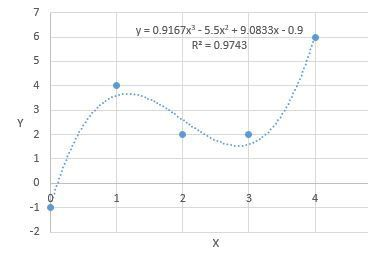#### polySolve(poly, n)

This function solves polynomial equation for a given n. The poly equation should be in the Dict format from the polyReg function.

Example

`[{x:0, y:-1}, {x:1, y:4}, {x:2, y:2}, {x:3, y:2}, {x:4, y:6}].toGrid().polyReg(3).polySolve(2)`

Returns: 2.6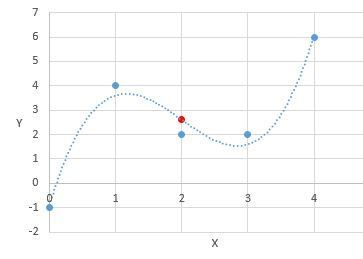#### polyDeriv(poly)

This function calculates the dervative for a polynomial equation in the Dict format from the polyReg function.

Example

`[{x:0, y:-1}, {x:1, y:4}, {x:2, y:2}, {x:3, y:2}, {x:4, y:6}].toGrid().polyReg(3).polyDeriv()`

Returns

```|   a0   |  a1   |  a2   | degree |
|  ----- | ----- | ----- |  ----- |
| 9.083  |  2.75 |  -11  |    2   |```

Where- y = a2 * x^2 + a1 * x + a0

This can be combined with the polySolve function to get the slope of the line at a given X.

```[{x:0, y:-1}, {x:1, y:4}, {x:2, y:2}, {x:3, y:2}, {x:4, y:6}].toGrid().polyReg(3).polyDeriv().polySolve(0.5)

= 4.271```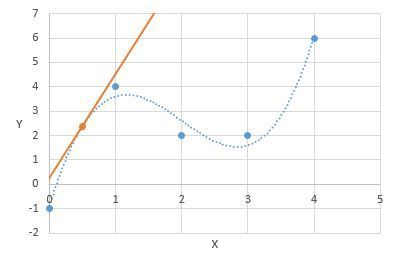#### polyInteg(poly)

This function calculates the integral for a polynomial equation in the Dict format from the polyReg function.

Example

`[{x:0, y:-1}, {x:1, y:4}, {x:2, y:2}, {x:3, y:2}, {x:4, y:6}].toGrid().polyReg(3).polyInteg()`

Returns

```|   a1  |  a2   |   a3   |   a4  | degree |
| ----- | ----- | ------ | ----- | ------ |
| -0.9  | 4.542 | -1.833 | 0.229 |    4   |```

Where- y = a4 * x^4 + a3 * x^3 + a2 * x^2 + a2 + a1 * x

This can be combined with the polySolve function to get the the area under a curve between two points.

```([{x:0, y:-1}, {x:1, y:4}, {x:2, y:2}, {x:3, y:2}, {x:4, y:6}].toGrid().polyReg(3).polyInteg().polySolve(3)) - ([{x:0, y:-1}, {x:1, y:4}, {x:2, y:2}, {x:3, y:2}, {x:4, y:6}].toGrid().polyReg(3).polyInteg().polySolve(1))

= 5.2```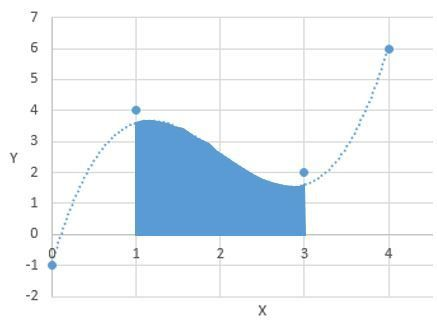#### quadSolve(poly)

This function solves a quadratic equation (second order polynomial) for zero.

Example

`[{x:0, y:-1}, {x:1, y:2}, {x:2, y:4}, {x:3, y:2}, {x:4, y:-1}].toGrid().polyReg(2).quadSolve()`

Returns

```|   v0  |   v1  |
| ----- | ----- |
| 0.254 | 3.746 |```

Where- v0 = (-b + SQRT(b^2 - 4ac))/2a- v1 = (-b - SQRT(b^2 - 4ac))/2a

This function can be used to solve the local minimum and maximum of a third degree polynomial

```[{x:0, y:-1}, {x:1, y:4}, {x:2, y:2}, {x:3, y:2}, {x:4, y:6}].toGrid().polyReg(3).polyDeriv().quadSolve()

= v0: 2.835, v1: 1.165

[{x:0, y:-1}, {x:1, y:4}, {x:2, y:2}, {x:3, y:2}, {x:4, y:6}].toGrid().polyReg(3).polySolve(2.835)

= 1.533

[{x:0, y:-1}, {x:1, y:4}, {x:2, y:2}, {x:3, y:2}, {x:4, y:6}].toGrid().polyReg(3).polySolve(1.165)

= 3.667

Local extremes: [{2.835, 1.533},{1.165, 3.667}]```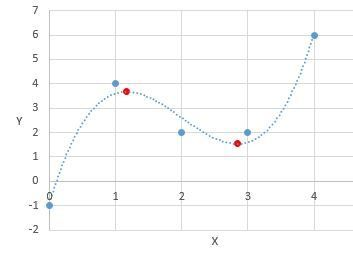Published by IoTWarez
Pricing options
 itwToolkitExtIoT Warez Toolkit FREE Also available via SkyArc Install Manager